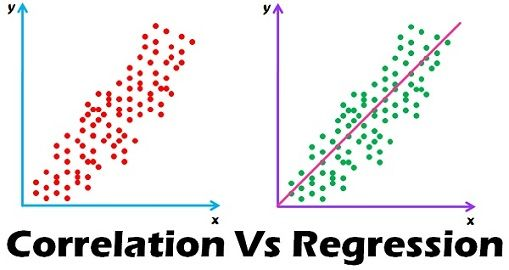#### Difference Between Correlation and Regression in StatisticsCorrelation and Regression are the two analysis based on multivariate distribution. A multivariate distribution is described as a distribution of multiple variables. Correlation is described as the analysis which lets us know the association or the absence of the relationship between two variables ‘x’ and ‘y’. On the other end, Regression…

Continue

Added by Asim Jana on March 8, 2018 at 3:30pm — 3 Comments

2018